# Program to check Tech Number in Java

In this tutorial you will learn about the Program to check Tech Number in Java and its application with practical example.

In this tutorial, we will learn to create a Java Program to check Tech Numbers in Java using Java programming.

## Prerequisites

Before starting with this tutorial we assume that you are best aware of the following Java programming topics:

• Java Operators.
• Basic Input and Output function in Java.
• Class and Object in Java.
• Basic Java programming.
• If-else statements in Java.
• For loop in Java.

## What is Tech Number.

A number is called a Tech number if an even number digit split into two halves(1/2) then square of the sum of those halves(1/2) is equal to the original number.

Example :->3025 So split 3025 into two halves

1. 30.
2. 25.
then = (30) +( 25)
= 55
3. =55*55.
4. =3025
So => 3025 is a tech number.

## Program to check Tech Number in Java

In this program we would create a java program to find given number is a  Tech number or not .First will take the input from the user and check whether it is Tech number or not. If it’s not then the program will show the output as it’s not a tech number.

## Output

Tech  Number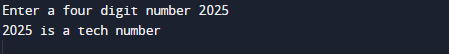Not a Tech Number.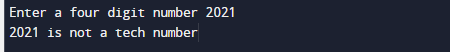In the above program, we have first declared and initialized a set variables required in the program.

• num,numberand no = it will hold entered number.
• fH and Lh =  it will hold first half and last half of a number.
• ct=  finding digit of a number.
• sum= sum of digit.

After that we take a number from user and find given number is a Tech number or not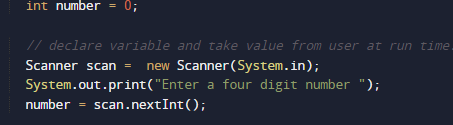after taking values we will pass number to function Tech() where we check multiple conditions as shown in image below.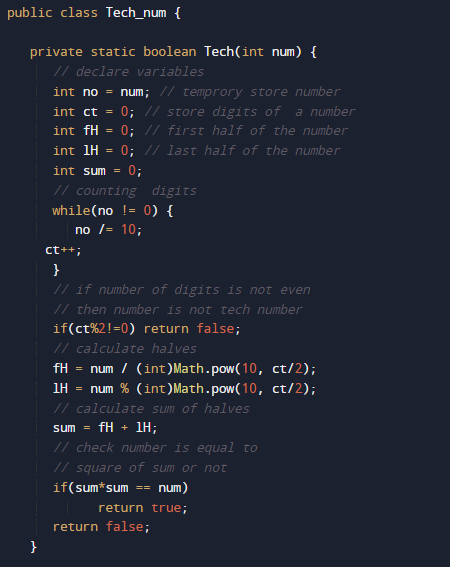check entered number is a four digit number or not. if given number is a four digit nmber then we break number into two half first two in hF variable and second two in lF variable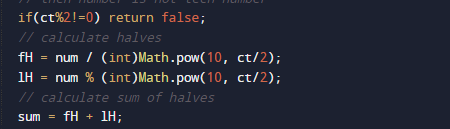and these two in variable sum where we find square of their addition.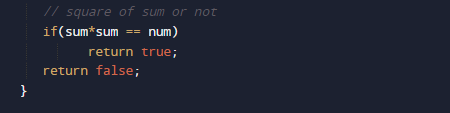and then compare square of sum equals to the given number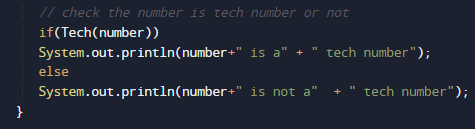then number is a Tech number otherwise it is not a tech number.

In this tutorial we have learn about the Program to check Tech Number in Java and its application with practical example. I hope you will like this tutorial.